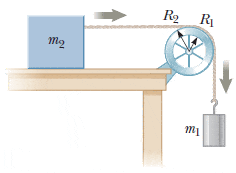# Difficult energy conservation/rotational energy problem

doneky

## Homework Statement

In the figure below, the hanging object has a mass of m1 = 0.405 kg; the sliding block has a mass of m2 = 0.825 kg; and the pulley is a hollow cylinder with a mass of M = 0.350 kg, an inner radius of R1 = 0.020 0 m, and an outer radius of R2 = 0.030 0 m. Assume the mass of the spokes is negligible. The coefficient of kinetic friction between the block and the horizontal surface isμk = 0.250. The pulley turns without friction on its axle. The light cord does not stretch and does not slip on the pulley. The block has a velocity of vi = 0.820 m/s toward the pulley when it passes a reference point on the table.## Homework Equations

KE = 1/2Iw^2
KE = 1/2mv^2
PE = mgh
Non-conservative work = (delta)KE + (delta)PE

## The Attempt at a Solution

The left side has negative work because of friction. On the right side, I put the KE of each as well as the potential energy (ONLY on m1, I believe?)

I think my only problem is the moment of inertia for the two pulleys. I don't understand how to implement the two radii. The answer shows that there is a combination of both. I have no idea why. Parallel axis theorem maybe?

Staff Emeritus